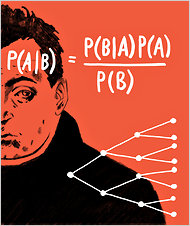Rags Srinivasan                                                http://iterativepath.wordpress.com

This article that reviews the new book on Bayesian probability uses this puzzle to explain the concept

Assume that you’re presented with three coins, two of them fair and the other a counterfeit that always lands heads. If you randomly pick one of the three coins, the probability that it’s the counterfeit is 1 in 3. This is the prior probability of the hypothesis that the coin is counterfeit. Now after picking the coin, you flip it three times and observe that it lands heads each time. Seeing this new evidence that your chosen coin has landed heads three times in a row, you want to know the revised posterior probability that it is the counterfeit. The answer to this question, found using Bayes’s theorem (calculation mercifully omitted), is 4 in 5. You thus revise your probability estimate of the coin’s being counterfeit upward from 1 in 3 to 4 in 5.

If you are interested in the math (which the article  says it mercifully omitted) on how they arrived at the answer of     here it is:

P(Counterfeit) = P(C)  =      (initial probability)P(we will get 3 heads in a row given it is the Counterfeit) = P(3H | C) = 1

You can see that if this is the counterfeit that always lands heads, probability of getting 2,3,4, etc heads in a row is 1 (it is certain).

P(Coin is not Counterfeit )  =1 -  =

P(Landing 3 heads in a row given it is not the Counterfeit) = P(3H | Not-C) = (1/2)^3 =

(chances of getting 1H is ½, next H is again ½ and the third time is ½, so we multiply)

(Note that this is also the same for getting any combination of heads and tails with a fair coin)

What the Times articles computes is

P(Counterfeit given we landed 3 heads in a row) = P(C|3H)We have values 2 of three factors on the right side of the equation. What we need is P(3H)

P(Landing 3 heads in a row) = P(Landing 3 heads in a row with the Counterfeit coin) +

P(Landing 3 heads in a row with Not-Counterfeit coin)

P(3H)      =  1 * (1/3)  + (1/8) * (2/3) =  5/12

So P(C|3H) =